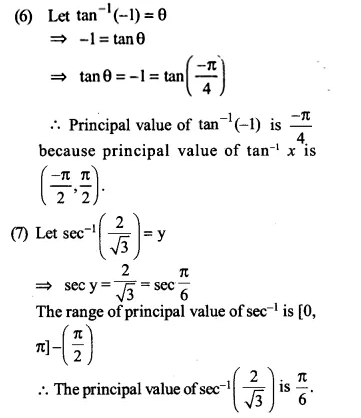# NCERT Solutions for Class 12 Maths Chapter 2 Inverse Trigonometric Functions Ex 2.1

Here we provide NCERT Solutions for Class 12 Maths Chapter 2 Inverse Trigonometric Functions Ex 2.1 for English medium students, Which will very helpful for every student in their exams. Students can download the latest NCERT Solutions for Class 12 Maths Chapter 2 Inverse Trigonometric Functions Ex 2.1 pdf, free NCERT solutions for Class 12 Maths Chapter 2 Inverse Trigonometric Functions Ex 2.1 book pdf download. Now you will get step by step solution to each question.

## NCERT Solutions for Class 12 Maths Chapter 2 Inverse Trigonometric Functions Ex 2.1

Ex 2.1 Class 12 Maths Question 1-10.
Find the principal values of the following:
(1)
(2)
(3)
(4)
(5)
(6)
(7)
(8)
(9)
(10)
Solution:
(1) Let$\sin ^{ -1 }{ \left( -\frac { 1 }{ 2 } \right) }$ = y
∴$sin\quad y=-\frac { 1 }{ 2 } =-sin\frac { \pi }{ 6 } =sin\left( -\frac { \pi }{ 6 } \right)$
the range of principal value of sin-1 isEx 2.1 Class 12 Maths Question 11-12.
Find the principal values of the following:
(11)
(12)
Solution:
(11)$\tan ^{ -1 }{ (1) } +\cos ^{ -1 }{ \left( -\frac { 1 }{ 2 } \right) } +\sin ^{ -1 }{ \left( -\frac { 1 }{ 2 } \right) }$
Now tan-1 (1) =$\frac { \pi }{ 4 }$
∴the range of principal value branch ofEx 2.1 Class 12 Maths Question 13.
If sin-1 x = y, then
(a) 0 ≤ y ≤ π
(b)
(c) 0 < y < π
(d)
Solution:
The range of principal value of sin is$\left[ -\frac { \pi }{ 2 } ,\frac { \pi }{ 2 } \right]$
∴ if sin-1 x = y then$-\frac { \pi }{ 2 } \le y\le \frac { \pi }{ 2 }$
Option (b) is correct

Ex 2.1 Class 12 Maths Question 14.
is equal to
(a) π
(b)
(c)
(d)
Solution:$\tan ^{ -1 }{ \sqrt { 3 } =\frac { \pi }{ 3 } ,\sec ^{ -1 }{ (-2) } } =\pi -\frac { \pi }{ 3 } =\frac { 2\pi }{ 3 }$
∴ Principal values of sec-1 is [0,π] –$\left\{ \frac { \pi }{ 2 } \right\}$$\tan ^{ -1 }{ \sqrt { 3 } - } \sec ^{ -1 }{ (-2) } =\frac { \pi }{ 3 } -\frac { 2\pi }{ 3 } =-\frac { \pi }{ 3 }$
Option (b) is correct

All Chapter NCERT Solutions For Class 12 Maths

—————————————————————————–

All Subject NCERT Solutions For Class 12

*************************************************

I think you got complete solutions for this chapter. If You have any queries regarding this chapter, please comment on the below section our subject teacher will answer you. We tried our best to give complete solutions so you got good marks in your exam.

If these solutions have helped you, you can also share ncertsolutionsfor.com to your friends.